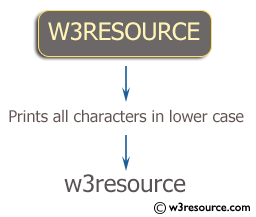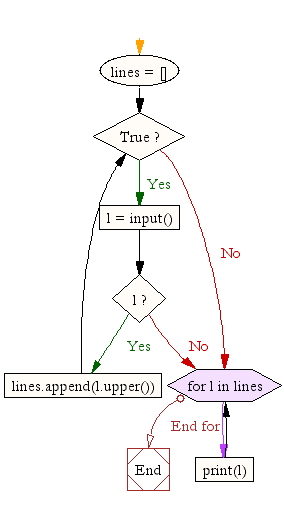﻿ Python Exercise: Sequence of lines as input and prints the lines as output in lower case - w3resource# Python Exercise: Sequence of lines as input and prints the lines as output in lower case

## Python Conditional: Exercise-12 with Solution

Write a Python program that accepts a sequence of lines (blank line to terminate) as input and prints the lines as output (all characters in lower case).

Pictorial Presentation:Sample Solution:

Python Code:

``````lines = []
while True:
l = input()
if l:
lines.append(l.upper())
else:
break;

for l in lines:
print(l)
```
```

Sample Output:

```w3resource
Python

W3RESOURCE
PYTHON
```

Flowchart:## Visualize Python code execution:

The following tool visualize what the computer is doing step-by-step as it executes the said program:

Python Code Editor:

Have another way to solve this solution? Contribute your code (and comments) through Disqus.

What is the difficulty level of this exercise?

Test your Python skills with w3resource's quiz

﻿

## Python: Tips of the Day

Returns a list with n elements removed from the beginning

Example:

```def tips_take(itr, n = 1):
return itr[:n]
print(tips_take([1, 2, 3], 5))
print(tips_take([1, 2, 3], 0))
```

Output:

```[1, 2, 3]
[]
```